Change List for this Rule

General Order 95

Appendix F

Typical Problems

Part I

Crossing Problem

A diagram including dimensions is shown on page F18. The data chosen for the crossing follow:

Data of Crossing

Circuits

Two 3phase 60,000 Volt power circuits. One metallic private Class C telephone circuit

Configuration

Power conductors of each circuit are in vertical planes on opposite ends of the crossarm. Private telephone circuit is in a horizontal plane.

Conductors

Power circuits are six No. 00 AWG, bare, stranded, harddrawn copper. Private telephone circuit is two No. 8 AWG, bare, solid, harddrawn copper, except in the crossing span where it is two No. 6 AWG, bare, solid, hardrawn copper.

Insulators

Porcelain, pin type, meeting the requirements of Rule 49.5A .

Ties

Annealed copper wire to comply with Rules 49.3B and 49.3C .

Pins

Power circuitswrought iron pipe (extra strong), 11/2 x 181/2, to comply with Rules 49.3B and 49.3-C . Assumed bonded in accordance with the requirements of Rule 53.4 .

Communication circuit - 11/2 x 9 locust

Crossarms

Power circuitsDouglas fir (dense), 43/4 x 53/4 x 12, 1.9 pin holes, 11/16 hole for through bolt.

Communication circuitDouglas fir (dense), 31/4 x 41/4 x 42, ll/2 pin holes, 11/16 hole for through bolt.

Crossarm Braces

Meeting the requirements of Rule 49.2C .

Poles

Western red cedar, round, butt treated

Span Length

Crossing span, 200 feet.

Adjacent spans, 150 feet

Construction Requirements

1.    Conductor Sags and Tensions

The conductors are assumed to be strung so that at normal conditions of 60°F and no wind the tension will be 35% of the ultimate tension of the conductors. From Chart No. 1, Page C6, it will be seen that under these conditions the No. 00 AWG conductor, for a 200 foot span, will have a sag of 1.0 foot (0.99 when calculated), and the No. 6 AWG conductor will have a sag of 0.90 foot (0.89 when calculated). These sags may be calculated by means of the following approximation formula: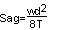Where w = conductor loading, pounds per lineal foot d = span length, feet T = assumed allowable conductor tensions at 60_F and no wind

For No. 00 AWG conductor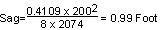For No 6 AWG conductor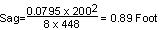Maximum conductor load to be met with a safety factor of not less than 2 as specified in Rule 44.1 will occur at the conditions of 25°F and an 8 pound wind ( Rule 43.2 ). Conductors which have been strung at the normal conditions stated above (60°F, no wind, and 35% ultimate tension) will have sags and tensions at the maximum loading conditions of 25°F and an 8 pound wind as indicated below. Maximum conductor sags will occur at the condition of maximum temperature, 130°F and also are shown in the following tabulation:

 #00 AWG #6 AWG Sag(Feet) Tension(Pounds) Sag(Feet) Tension(Pounds) Ultimate Conductor Tension (See Appendix B, Table 18) - 5,925 - 1,280 35% Ultimate at 60°F, No Wind 0.99 2,074 0.89 448 25°F, 8 Lb Wind 0.95 2,605 1.18 570 130°F, No Wind 1.78 1,157 1.55 256

From the foregoing it will be seen that by stringing the conductors to 35% of ultimate tension at 60°F and no wind, the safety factor of the conductors at maximum loading (25°F, 8 lb wind) is somewhat greater than the minimum of 2 required by Rule 44.1

Lesser sags than those shown above may be used, provided conductor tension, at maximum loading condition specified in Rule 43 , does not exceed 50% of the ultimate tension of the conductor. The rules, of course, do not prevent the use of greater sags than are calculated above.

2.    Conductor Clearance from Center Line of Pole

Minimum clearances specified in Table 1, Case 8 and Rule 54.4D2 and the clearances assumed for the purposed of this problem are as follows:

 Minimum Used 60,000 Volt circuits 21.5 5 6 Communications circuit 15 18

3.    Conductor Separation

Table 2, Case 12, Column H modified by Rule 54.4Clc , permits a vertical separation of not less than 36 inches between the conductors of a 60,000 Volt circuit in vertical configuration. For this problem a separation of 56 is used.

The minimum separation between the level of the lowest supply conductor and the communication circuit is 72 inches ( Table 2, Case 8, Column H ). For the problem, a separation of 96 inches between crossarm centers is used.

4.    Clearances of Conductors Above Crossarms

The minimum clearance of a 60,000volt conductor from the surface of a crossarm is required (by Table 1, Case 9, Column F ) to be at least 1/4 of the pin spacing specified in Table 2, Case 15, Column H , which would be a minimum clearance of 9 inches. For this problem, an 181/2 inch pin is used which, with its insulator, places the conductor 14 inches above the crossarm.

5.    Conductor Clearances Above Highway, Pole Lines and Railroad Tracks

The poles supporting the crossing span are 55 feet in length, set 7 feet (Rule 49.1C ) in the ground. From dimensions of the pole framing diagram the distance of the private telephone circuit above ground is 28 4. For this problem, a common elevation has been assumed for the ground line, the railroad tracks and the highway.

The sag of the communication conductors in the crossing span is approximately 11 inches at 60°F and 19 inches at 130° F. Since the allowable variation of 5% for temperature, applied to the ground clearance of 27 5 (28 4 - 11), is 1 4, which is greater than the difference between the sags at 60°F (11) and at 130°F (19), the clearances may be determined at 60°F for all conditions. In the diagram, Page F18 , the distances from supporting pole C to the various objects crossed over by the conductors are as follows:

 Telephone pole line 37 6 Highway (center) 60 0 Telegraph pole line on RR r/w 97 6 Railroad Tracks (center) 138 9 Railroad Signal pole line 180 0

The total length of crossing span is 200 feet. Therefore, the clearance at 60°F of the private communications circuit above the telephone lead at point of crossing is obtained as follows:

Clearance point distance from Pole C is 37 6.

At 37 6, or 18.8% of the span, the sag is equivalent to 61% of the center sag (see Chart No. 9 on Page C14 ), or 0.61 x 11 = 7 sag.

Therefore, the clearance equals:

28 4 - (7 + 24) = 3 9 clearance.

The minimum required clearance as given in Table 2, Case 3, Column C is 2 feet.

In a like manner the clearances, at 60°F, of the private communication circuit conductors at the other points of crossing are as follows:

 Points of crossing Clearances Minimum by Rule Highway (center) 27 7 18 0 Telegraph pole line 3 5 2 0 Railroad Tracks (center) 27 7 25 0 Railroad Signal pole line 6 0 2 0

6.    Insulators

In addition to the electrical requirements set forth in Rules 55 and 104 , the insulators supporting the supply and communication conductors shall have safety factors (mechanical) of 3 and 2, respectively.

7.    Pins, Ties and Conductor Fastenings

Ties used in connection with pintype insulators shall conform to Rule 49.3 . In this problem a No. 4 and No. 8 annealed copper wire are used for the No. 00 and No. 8 circuits involved.

Pins used in connection with pintype insulators shall have sufficient strength to withstand the tension in the conductor. In the case under discussion wrought iron pipepins of the dimensions and construction indicated below are to be employed for the power conductors.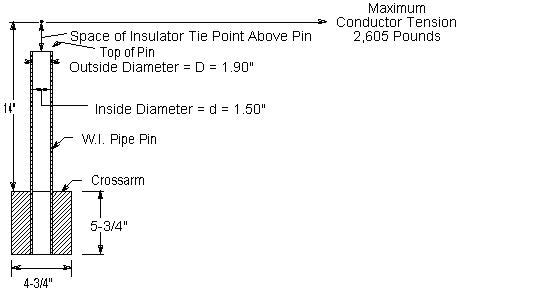Bending moment (at crossarm) M = 2,605 x 14 = 36,470 poundinches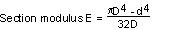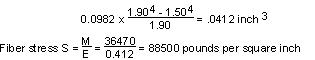Assuming that the ultimate fiber stress of wrought iron is 48,000 pounds per square inch, a single pin is not sufficient, as it provides a safety factor of 0.542,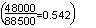for an assumed tension of 2,605 pounds in the conductor at maximum loading. Since a safety factor of unity (Rule 47.3 ) is required, two pins are necessary and therefore double crossarms, pins and insulators are used on the poles supporting the crossing span.

Locust pins are to be used in this case for the private telephone conductors. Although a 11/2 inch locust pin would be sufficient to withstand the conductor tension of 570 pounds with a safety factor of at least unity, as required by Rule 47.2 , care would be necessary to provide sufficient strength in the conductor fastenings. In this problem, the private telephone conductors are considered to be deadended at the ends of the crossing span.

Power Circuits

The point of maximum bending moment will be at the crossarm through bolt attaching the arm to the pole, at which point the cross section of the arm is reduced by the amount of the bolt hole. Crossarms supporting the 60 kV wires are to be Douglas fir, dense, dimensions 43/4 x 53/4 x 12, bored as illustrated below.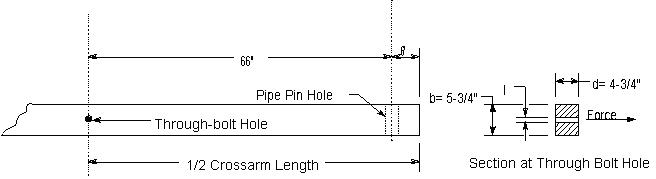The section through the arm and the method of computing the fiber stress is shown below.

Longtime loading: Since longitudinal conductor loads are normally balanced, longtime horizontal loading of the power circuit crossarms need not be considered.

Single arm, Maximum loading, 25°F and an 8 lb wind

Bending moment = 2,605 x 66 = 171,930 poundinches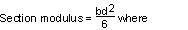b = 5.75  0.69 = 5.06

d = 4.75

s = 11/16 = 0.69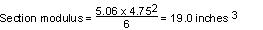Fiber Stress = Bending moment divided by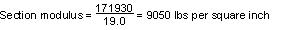As the allowable value for modulus of rupture in bending under maximum loading conditions is 6,300 lbs per sq. in. (see Table 5 , Rule 48.1 ), a single crossarm of the size chosen provides a safety factor of only 0.70 for the assumed load at maximum loading conditions, whereas the provisions of Rule 47.3 require a safety factor of unity. Double arms will, therefore, be used in this problem to meet the strength requirements applicable to crossarms at end supports of crossings. Double crossarm construction of this type with separation maintained by space bolts is assumed to have a horizontal strength equivalent to 130% of the sum of the strengths of two single crossarms acting independently.

Maximum loading, 25°F and an 8 lb wind

Bending moment = 2,605 x 66 = 171,930 poundinches

Single arm section modulus (same as previously calculated) = 19.0 inches

Double arm section modulus = 19.0 x 2 x 1.3 = 49.4 inches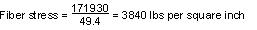As the allowable modulus of rupture for shorttime loading is 6,300 lbs per sq. in. then the double crossarms under these conditions will provide a safety factor of 1.91, which meets the unity safety factor required by Rule 47.3.

Private Communication Circuit

At the crossing span, double crossarms are used on account of deadend construction due to change of conductor size. Current practice provides for this method of construction although a singlearm has sufficient strength as is found form the following calculations of modulus of rupture under the two limiting conditions of loading: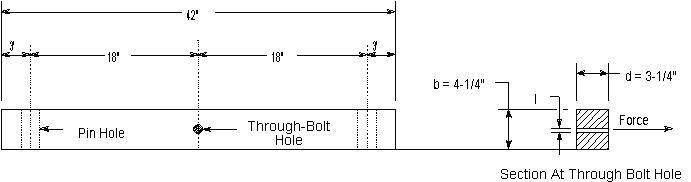Bending moment = 448 x 18 = 8,064 poundinches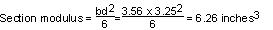where d = 3.25

s = 0.69

b = 4.25  0.69 = 3.56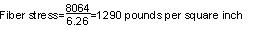The allowable value for modulus of rupture in bending is 0.55 x 6,300 = 4,465 pounds per square inch and therefore with a single arm the factor of safety under conditions of longtime loading is 2.69.

Bending moment = 570 x 18 = 10,260 poundinches

Section modulus = 6.26 inches3 (as per calculations above)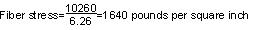The allowable value for modulus of rupture in bending, under maximum loading conditions, is 6,300 pounds per sq. in., therefore a single arm provides a safety factor of 3.84 under these maximum loading conditions.

9.    Crossarms - Vertical Loads

The vertical load on crossarms, where supports are approximately at the same elevation, is due to the vertical load of conductors in each adjacent span plus 200 pounds at the outer pin position. In the problem under consideration, the conductor supports on the crossing poles (C and D) are at the same elevation, and the supports at the adjacent poles (B and E) are 4.5 feet lower in elevation, which difference in elevation is greater than the normal sag. Then the conductor loading on a crossing span support would be onehalf the weight of the conductor of the crossing span plus onehalf the conductor weight of a hypothetical span, the curve of which passes through the points of support.

Half the length of the hypothetical span may be calculated as follows: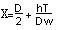Where

X = 1/2 the hypothetical span in feet.

D = horizontal distance between supports in feet.

h = difference in elevation of supports in feet.

T = conductor tension in pounds.

w = weight of conductor in pounds per foot.

The total crossing supportload is calculated as follows: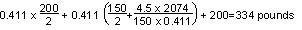The bending moment is: 334 x 66 = 22,040 poundinches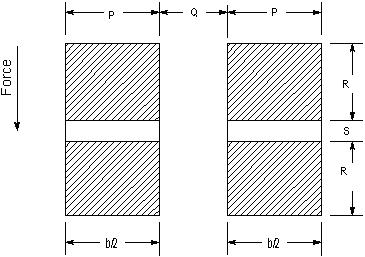The method of calculating the unit fiber stress of the double crossarms acting as a simple beam is as follows: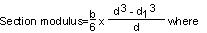b = P + P = 9.50

d = R + S + R =  5.75

d1 = S =  0.69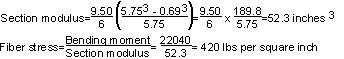As the allowable modulus of rupture in bending is 0.55 x 6,300 lbs per sq. in. or 3,465 lbs per sq. in. (see Table 5 ), the double crossarms of the size chosen provide a safety factor of 8.2.

The fiber stress in the double crossarms of the private telephone circuit, similarly calculated, is found to be 196 lbs per sq. in. These arms obviously meet the strength requirements for vertical loads on crossarms.

Shear, compression and torsion stresses are not considered in this problem as they are negligible and likewise the effect of reduction of cross section due to bolt holes is not considered except for the through bolt holes.

10.    Poles

The crossing poles are western red cedar and their dimensions are as follows:

 Length 55 feet Height above ground 48 feet Circumference at top 28 inches Diameter at top 8.9 inches Circumference at ground line 49.0 inches Diameter at ground line 15.6 inches

Distance from ground line to conductors supported is given as follows:

 Top supply conductors 48 9 Middle supply conductors 43 3 Lower supply conductors 37 9 Private telephone conductors 28 4

Ground level at base of pole is considered to be at the same elevation as top of rail.

Dimensions of adjacent poles B and E are:

 Length 50 feet Height above ground 43.5 feet Circumference of top 28 .0 inches Diameter of top 8.9 inches Circumference at ground line 47.0 inches Diameter at ground line 15.0 inches

11.    Transverse Load on Crossing Poles C and D

The moment at the ground due to an 8 pound wind pressure on conductors is: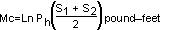Where:

 L = Height of conductors above ground in feet n = Number of wires S1 and S2 = Length of crossing and adjacent spans, respectively Ph = Horizontal load per lineal foot due to an 8 pound wind pressure on projected area of wire Ph = 0.276 pounds per lineal foot for 00 AWG bare, stranded copper = 0.108 pounds per lineal foot for 6 AWG bare, solid copper = 0.085 pounds per lineal foot for 8 AWG bare, solid copper Mc0 = Moment due to pressure on top supply conductors Mc1 = Moment due to pressure on middle supply conductors Mc2 = Moment due to pressure on lower supply conductors Mc3 = Moment due to pressure on telephone conductors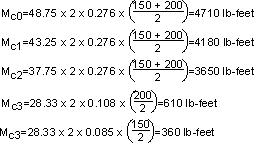Total Moment due to Wind pressure on conductors =    13,510 lb-feet

The moment at the ground due to an 8 pound wind pressure on the pole is: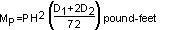Where:

 Mp = Moment due to wind pressure on pole P = Pressure in lbs per sq. ft. on projected area of pole (8 lbs/sq. ft.) H = Height of pole above ground in feet (48) D1 = Diameter of pole at ground in inches (15.6) D2 = Diameter of pole at top in inches (8.9)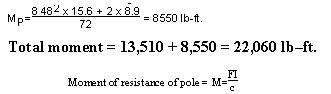Where: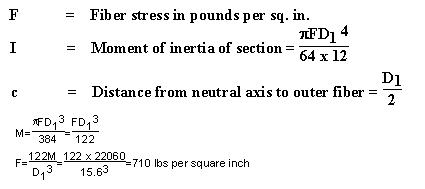The allowable fiber stress for western red cedar poles to provide a factor of safety of 4 is 1,500 pounds per sq. in., hence the crossing poles are not required to be side guyed since they have a factor of safety of 8.5 for transverse load.

12.    Side Guying

If side guying were required for the crossing poles C and D the method of computing the same would be as follows:

Side guys are designed to take the entire transverse load of the pole, the pole acting merely as a strut.

The transverse force acting on the poles will be due to wind pressure on poles C and D and the transverse wind pressure on the conductors supported. The length of conductor used in computing this transverse force will be equal to onehalf the distance between the guyed poles C and D, plus onehalf the length of the span adjacent to these poles.

The total wind pressure is computed as follows:

On Conductors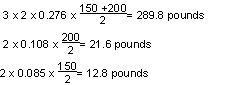On Pole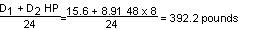Total Wind Pressure = 716.4 pounds

The total moment on the poles is the same as developed for Transverse load on poles which was 22,060 poundfeet.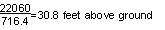A side guy could not be attached at this center of load and provide the required clearances from the communication line; therefore, for construction purposes the guy is assumed attached just below the lowest supply crossarm at a distance of 36 feet above ground.0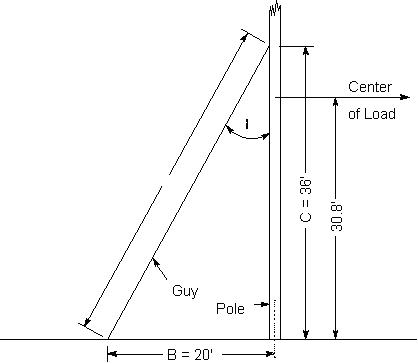Let Mt = Total moment on pole = 22,060 poundfeet C = Height of guy attachment above ground = 36 feet (assumed) B = Distance of guy anchor from base of pole = 20 feet T = Tension in guy wire in pounds A = Length of guy =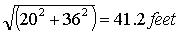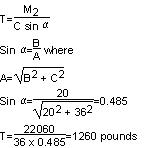The specified safety factor for guys (Table 4 , Rule 44.1 ) is 2 and, therefore, a guy having an ultimate strength of not less than 2,520 pounds is required. One 1/4 inch SiemensMartin or a 5/16 inch common galvanizedsteel strand would meet the requirements for transverse load.

13.    Longitudinal Load on Crossing Poles C and D

Rule 47.3   provides that crossing structures shall withstand at all times with a safety factor of unity the unbalanced stress due to the combined pull toward the crossing of onethird of the total number of conductors supported, the pull in each such conductor being taken as the tension due to the specified loading.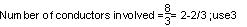Location of conductors resulting in maximum load - two on top arm and one on next arm below

Bending moment:

2 x 2,605 x 48.75 =    254,000 poundfeet

1 x 2,605 x 43.25 =    112,600 poundfeet

Total Moment =    366,600 poundfeet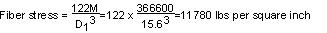The allowable value of modulus of rupture under this load is 6,000 lbs per square inch, hence poles C and D must be head guyed for longitudinal load.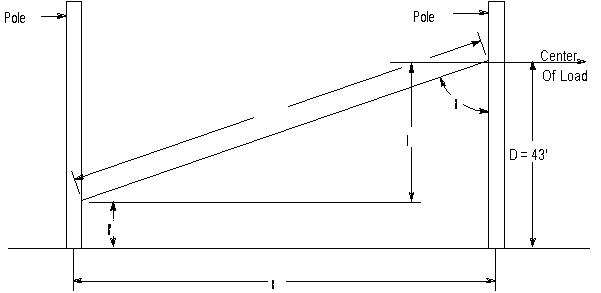The head guy should be attached approximately at the normal center of load, therefore:

The bending moment under full longitudinal load would be:

 48.75 x 2 x 2,605 = 254,000 poundfeet 43.25 x 2 x 2,605 = 225,300 poundfeet 37.75 x 2 x 2,605 = 196,700 poundfeet 28.33 x 2 x 570 = 32,300 poundfeet Total moment = 708,300 poundfeet

The total longitudinal load would be:

 3(2 x 2,605) = 15,630 pounds 2 x 570 = 1,140 pounds Total wire tensions = 16,770 pounds

Therefore, the center of longitudinal load is: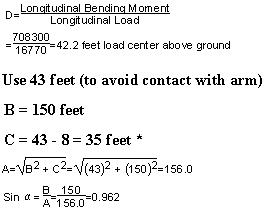* Lower end of guy assumed 8 feet above ground on Poles A and E.

A guy attached at a point 43 feet above ground on pole C or D and at a point 8 feet above ground on pole B or E, respectively, would be required to withstand a load of: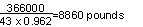In this case, a 9/16 inch common, 7/16 inch SiemensMartin, or 3/8 inch highstrength guy strand would meet the requirements of Rule 47.3. The horizontal load transmitted to pole B or E by such a head guy would be:

8,860 x sin ð = 8,860 x 0.962 = 8,520 pounds

The longitudinal moment on pole B and E would be:

8,520 x 8 = 68,160 poundfeet

and the fiber stress developed in pole B or E by the tension of 9,070 pounds in the head guy would be: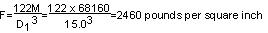Poles B and E would, therefore, be adequate to hold the contemplated guy tension with a safety factor of unity as required by Rule 47.3.

Note:      Revised February 5, 2014 by Decision No. 14-02-015.